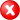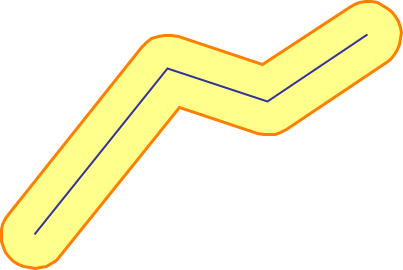#Boost C++ Libraries

...one of the most highly regarded and expertly designed C++ library projects in the world.

##### buffer (with strategies)

Calculates the buffer of a geometry.

###### Description

The free function buffer calculates the buffer (a polygon being the spatial point set collection within a specified maximum distance from a geometry) of a geometry.

###### Synopsis

```template<typename GeometryIn, typename MultiPolygon, typename DistanceStrategy, typename SideStrategy,
typename JoinStrategy, typename EndStrategy, typename PointStrategy>
void buffer(GeometryIn const & geometry_in, MultiPolygon & geometry_out, DistanceStrategy const & distance_strategy,
SideStrategy const & side_strategy, JoinStrategy const & join_strategy, EndStrategy const & end_strategy,
PointStrategy const & point_strategy)```

###### Parameters

Type

Concept

Name

Description

GeometryIn const &

Any type fulfilling a Geometry Concept

geometry_in

A model of the specified concept

MultiPolygon &

A type fulfilling the MultiPolygon Concept

geometry_out

output multi polygon (or std:: collection of polygons), will contain a buffered version of the input geometry

DistanceStrategy const &

A strategy defining distance (or radius)

distance_strategy

The distance strategy to be used

SideStrategy const &

A strategy defining creation along sides

side_strategy

The side strategy to be used

JoinStrategy const &

A strategy defining creation around convex corners

join_strategy

The join strategy to be used

EndStrategy const &

A strategy defining creation at linestring ends

end_strategy

The end strategy to be used

PointStrategy const &

A strategy defining creation around points

point_strategy

The point strategy to be used

Either

`#include <boost/geometry.hpp>`

Or

`#include <boost/geometry/algorithms/buffer.hpp>`

###### Strategies

The 5 strategies give the user control to the generated buffer

• convex corners can be rounded or sharp
• line-ends can be rounded or flat
• distance can be symmetric or assymetric, and positive or negative
• around points the shape can be circular or square
• the sides can be controlled (currently there is only one option provided)

By specifying a negative distance for the distance_strategy, for the (multi) polygon case, the polygon will be smaller (also known as deflate). The distance cannot be 0.

The next figure shows where in the generated buffer the strategies have effect###### Conformance

The function buffer implements function Buffer from the OGC Simple Feature Specification.

It is conformant if used with the following combination of strategies: join_round, end_round, distance_symmetric, point_circle, side_straight

###### Supported geometries

2D Cartesian

PointSegmentBoxLinestringRingPolygonMultiPointMultiLinestringMultiPolygon###### Examples

Shows how the buffer algorithm can be used to create a buffer of a linestring, a multi point, a multi polygon

```#include <boost/geometry.hpp>
#include <boost/geometry/geometries/point_xy.hpp>
#include <boost/geometry/geometries/geometries.hpp>

int main()
{
typedef double coordinate_type;
typedef boost::geometry::model::d2::point_xy<coordinate_type> point;
typedef boost::geometry::model::polygon<point> polygon;

// Declare strategies
const double buffer_distance = 1.0;
const int points_per_circle = 36;
boost::geometry::strategy::buffer::distance_symmetric<coordinate_type> distance_strategy(buffer_distance);
boost::geometry::strategy::buffer::join_round join_strategy(points_per_circle);
boost::geometry::strategy::buffer::end_round end_strategy(points_per_circle);
boost::geometry::strategy::buffer::point_circle circle_strategy(points_per_circle);
boost::geometry::strategy::buffer::side_straight side_strategy;

// Declare output
boost::geometry::model::multi_polygon<polygon> result;

// Declare/fill a linestring
boost::geometry::model::linestring<point> ls;
boost::geometry::read_wkt("LINESTRING(0 0,4 5,7 4,10 6)", ls);

// Create the buffer of a linestring
boost::geometry::buffer(ls, result,
distance_strategy, side_strategy,
join_strategy, end_strategy, circle_strategy);

// Declare/fill a multi point
boost::geometry::model::multi_point<point> mp;

// Create the buffer of a multi point
boost::geometry::buffer(mp, result,
distance_strategy, side_strategy,
join_strategy, end_strategy, circle_strategy);

// Declare/fill a multi_polygon
boost::geometry::model::multi_polygon<polygon> mpol;
boost::geometry::read_wkt("MULTIPOLYGON(((0 1,2 5,5 3,0 1)),((1 1,5 2,5 0,1 1)))", mpol);

// Create the buffer of a multi polygon
boost::geometry::buffer(mpol, result,
distance_strategy, side_strategy,
join_strategy, end_strategy, circle_strategy);

return 0;
}
```

###### The linestring case###### The multi_point case###### The multi_polygon case